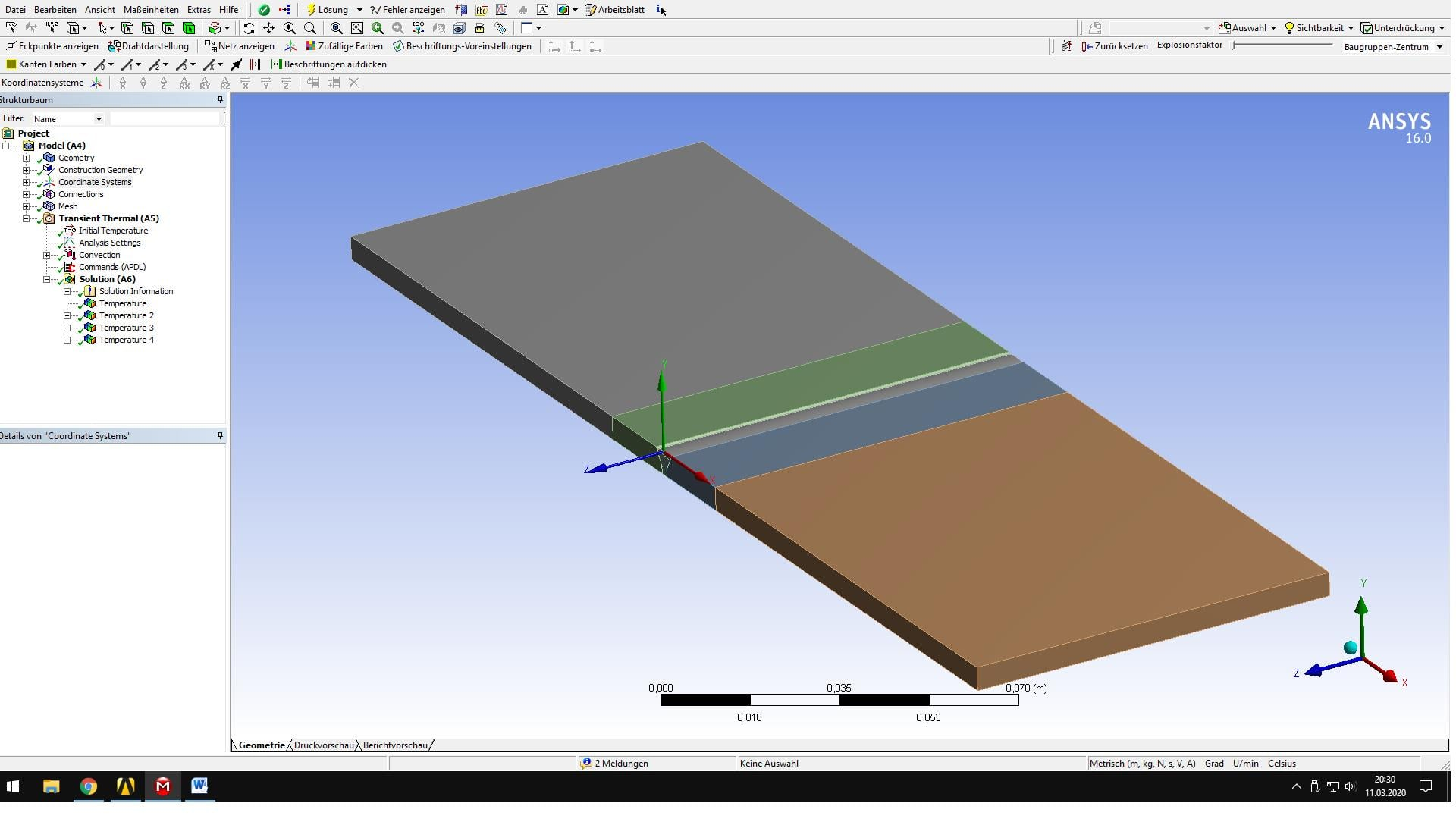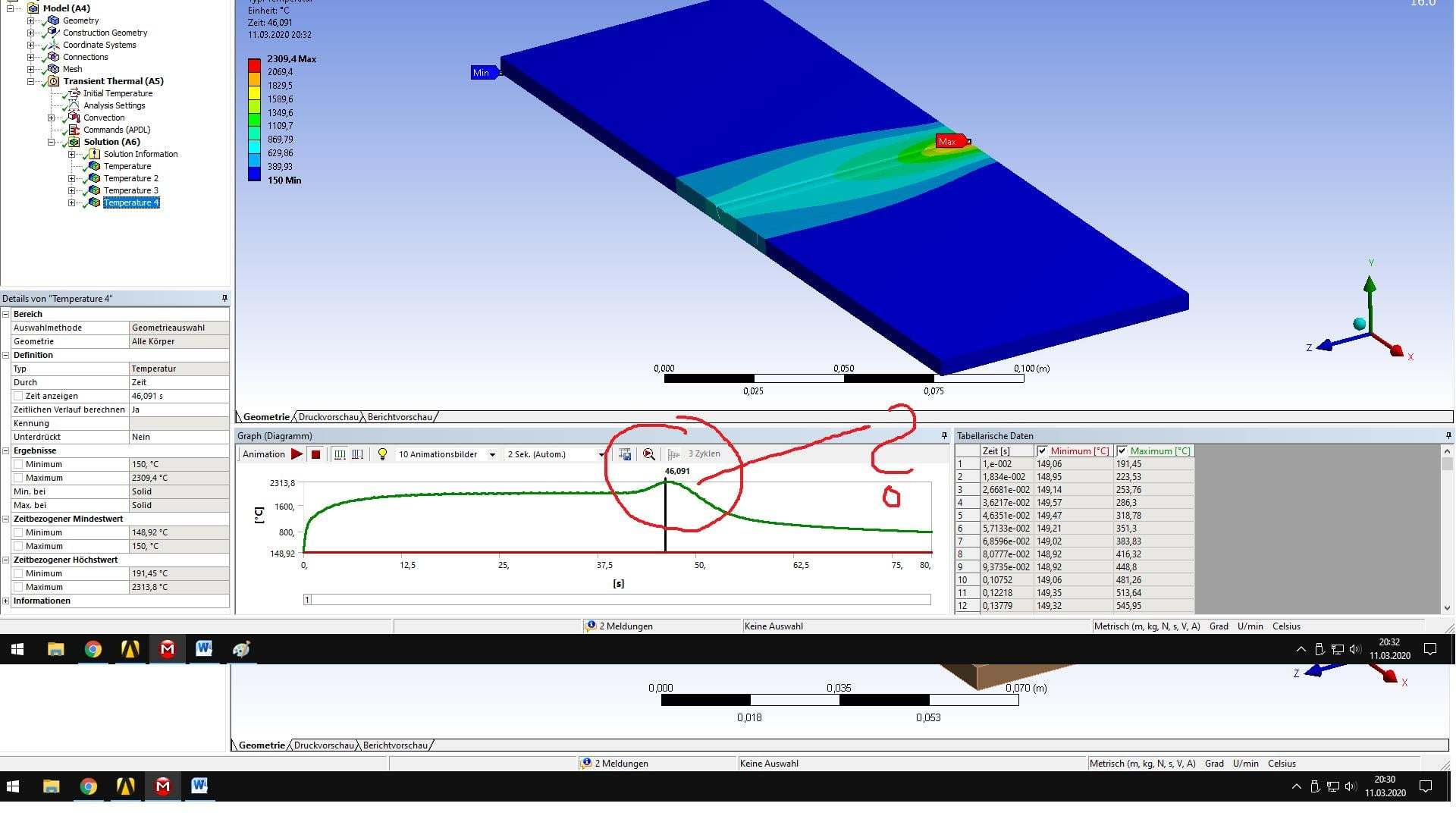# Goldak double ellipsoid laser welding

Hi,

I use an APDL command snippet to achieve a double ellipsoid according to Goldak equation, but the Temperature rise at the end of the welding line is strange and artificial.

The APDL snippet is:

CMSEL,ALL

*GET,EMAX,ELEM,,NUM,MAX

*GET,EMIN,ELEM,,NUM,MIN

ALLSEL

TIME_WELD=80

DT=1

A=0.003

B=0.003

C1=0.004

C2=0.016

TAU=0

FF=0.6

FR=1.4

Q=1000

VEL=1.73e-3

NPT=TIME_WELD/DT

NROPT, FULL

*DO,i,1,NPT,1

WTIME=(i)

TIME,WTIME

HCENTER=VEL*WTIME

*DO,jj,EMIN,EMAX,1

X=CENTRX(jj)

Y=CENTRY(jj)

Z=CENTRZ(jj)

CSI=-Z+VEL*(TAU-WTIME)

*IF,Z,GT,HCENTER,THEN

C=C1

F=FF

*ELSE

C=C2

F=FR

*ENDIF

PART1=(6*(3**0.5)*F*Q)/(A*B*C*3.14*(3.14**0.5))

PART2=(exp(-3*(X/A)**2))*(exp(-3*(Y/B)**2))*(exp(-3*(CSI/C)**2))

QF=PART1*PART2

BFE,jj,HGEN,,QF

*ENDDO

SOLVE

*ENDDO

CMSEL,ALL

BFEDELE,ALL,ALL

ALLSELThis is when the Laser spot leaves the plate.

Has anyone a suggestion what went wrong?

Best regards

Holly

• I met the same difficulty. Have you solved the problem?I guess it's because when the reservoir leaves the solid, there's no element in the first half that causes the heat flux to change in the second half, and I don't know if I'm right.

• Hi,

lost my password and therefore can't reactivate my account.

Unfortunately, I haven't solved the problem. I think you are right, but I'm not skilled enough to implement it in the code.

Do you have any suggestions to fix it in the code?

• Hi,

I think this problem arises because you use BFE to apply loads to elements, and maybe you should load the FLUX on nodes use BF,I‘m’ trying it now,If you have any progress, please tell me , thank you!

• Hi,

did you solve the issue? I wasn't successful.

• Hello,

If you are not finding a reply here, please create a new post with your detailed question and we will be happy to help.

Thanks.

Karthik